# M7-S9: The Photoelectric Effect and The Quantum Model of Light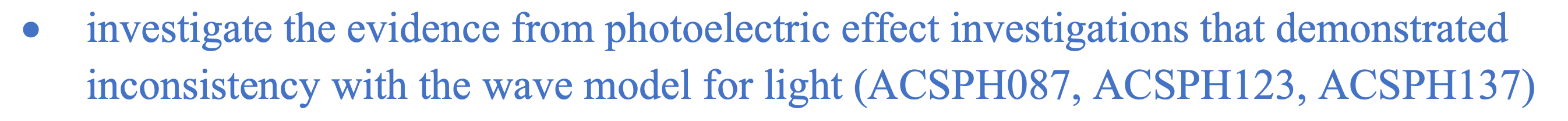#### Hertz’s observation of photoelectric effect

The photoelectric effect was first documented in 1887 by the German physicist Heinrich Hertz and is therefore sometimes referred to as the Hertz effect. While working with a spark-gap transmitter, Hertz discovered that upon absorption of certain frequencies of light, substances would give off a visible spark.

The classical picture underlying the photoelectric effect was that the atoms in the metal contained electrons, that were shaken and caused to vibrate by the oscillating electric field of the incident radiation. This theory was derived from Maxwell’s electromagnetic wave theory.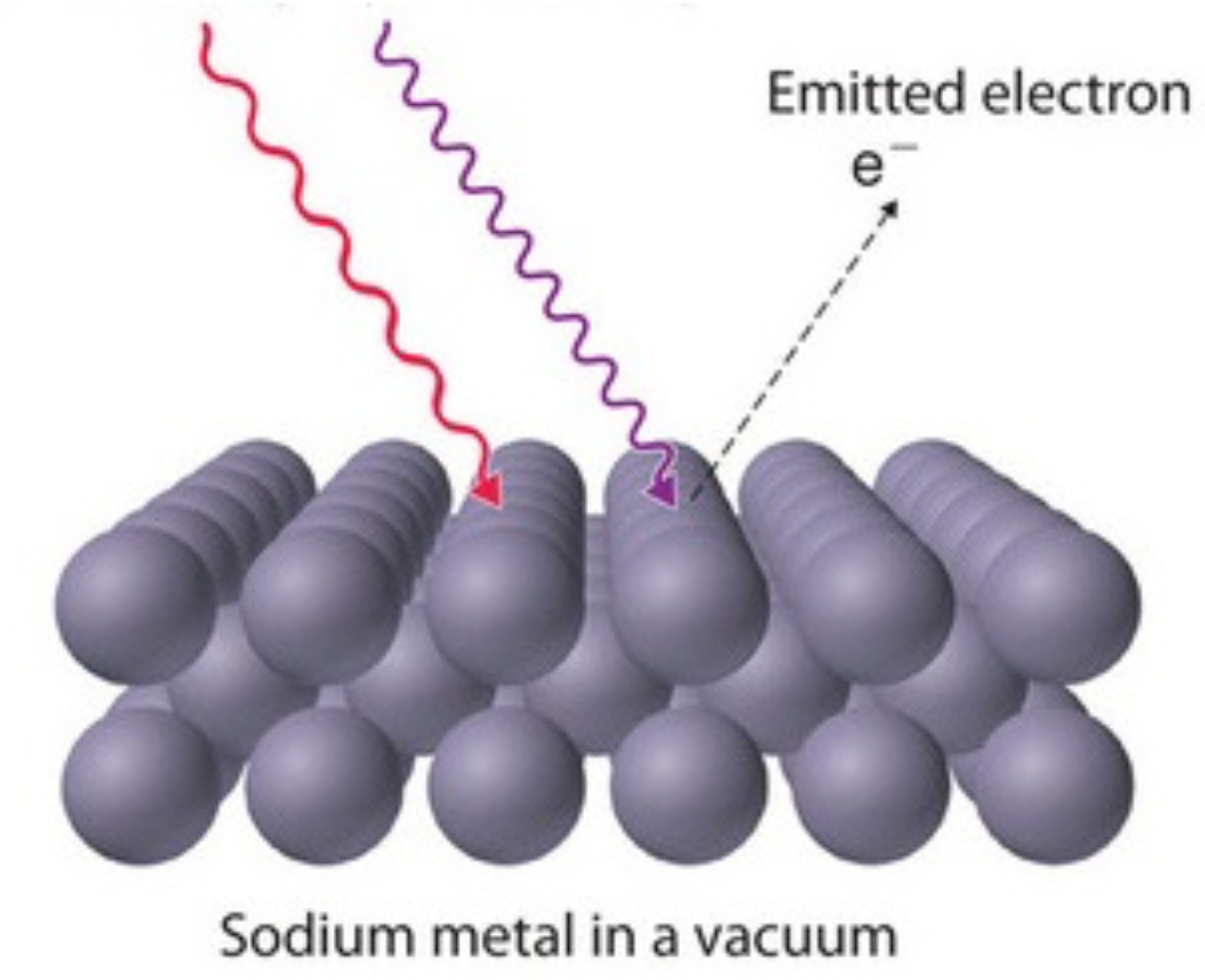Eventually some of these electrons would be shaken loose, and would be ejected from the cathode. It is worthwhile considering carefully how the number and speed of electrons emitted would be expected to vary with the intensity and color of the incident radiation along with the time needed to observe the photoelectrons.

What the wave model of light predicts

• Increasing the intensity of radiation would shake the electrons more violently, so one would expect more to be emitted, and they would shoot out at greater speed, on average.
• Increasing the frequency of the radiation would shake the electrons faster, so it might cause the electrons to come out faster. For very dim light, it would take some time for an electron to work up to a sufficient amplitude of vibration to shake loose.

Lenard's experimental results supported against the wave model of light

In 1902, Hertz's student, Philipp Lenard, studied how the energy of the emitted photoelectrons varied with the intensity of the light.

• He used a carbon arc light and could increase the intensity a thousand-fold. Light was incident on the positive electrode (cathode)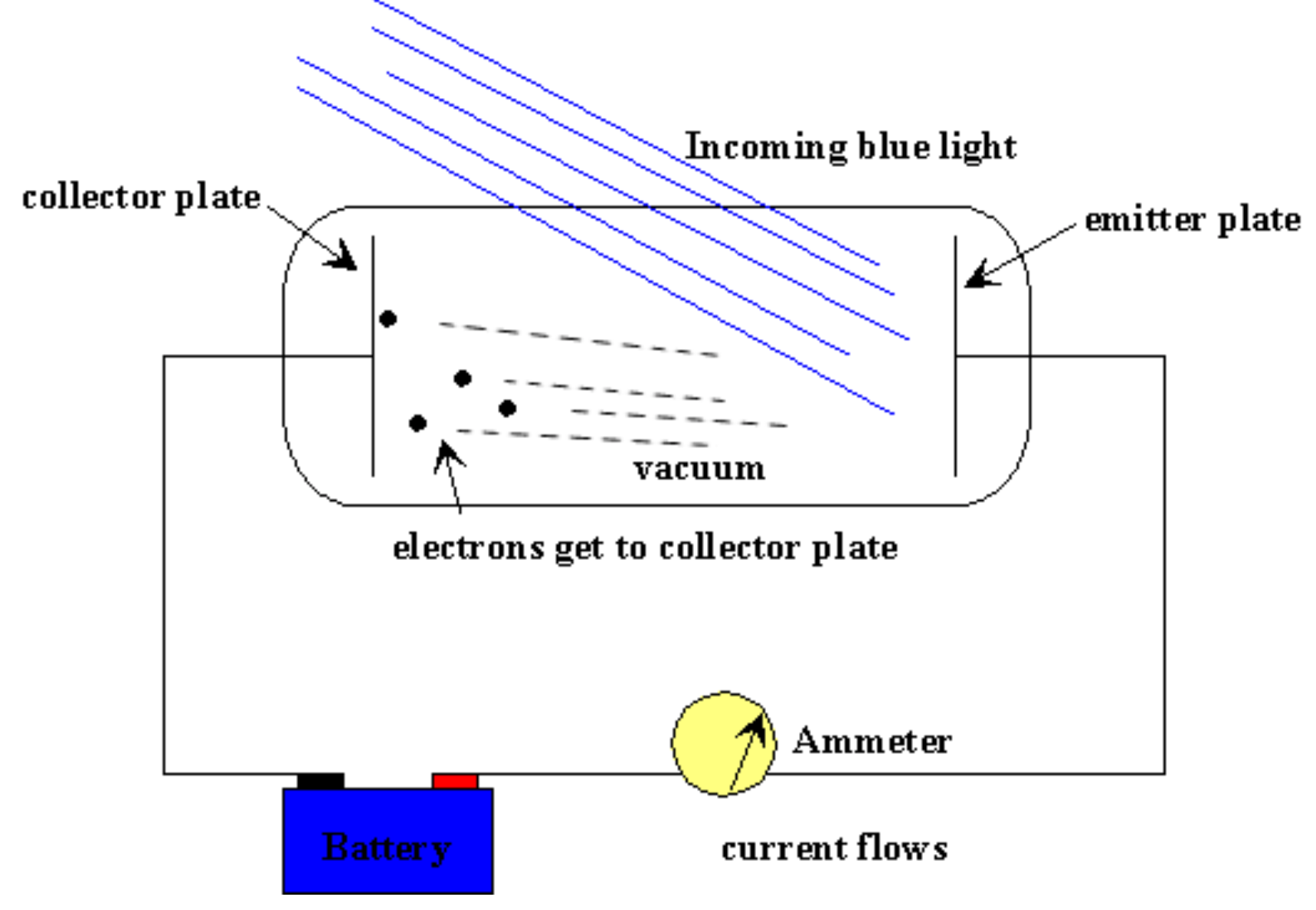Measurement of number of electrons

• The ejected electrons hit another metal plate (anode), the collector, which was connected to the cathode by a wire with a sensitive ammeter.

Ejected electrons flow through the wire from the anode back to the cathode. When an ammeter is placed in between, the magnitude of current or electron flow per unit time can be measured.

Measurement of energy of electrons

• To measure the energy of the ejected electrons, Lenard charged the collector plate negatively, to repel the electrons coming towards it. Thus, only electrons ejected with enough kinetic energy to get up this potential hill would contribute to the current. The voltage required to stop the current flow is the stopping voltage

Why couldn’t Huygens’ wave model of light explain the photoelectric effect?

• The relationship between light’s intensity and electron energy was not experimentally supported as greater intensity did not increase the stopping voltage.
• From last section: the interaction between wave and particle was poorly understood. The ability of a wave to physically move a particle (e.g. electron) was incomprehensible.#### Einstein’s particle model provided a valid interpretation of photoelectric effect

In 1905, Einstein provided a simple explanation of Lenard’s results by borrowing Planck’s quantum theory.

• Einstein proposed that when the incoming radiation is incident on an object, the energy transfer occurs between one quantum (one unit of energy) and one electron. He defined this quantum unit a He stated that a photon would transfer the entirety of its energy to an electron or none at all; a photon cannot transfer its energy partially.

Quantum vs photon – what is the difference?

A quantum is typically a generic term used to describe the smallest unit of a quantity including electromagnetic radiation. A photon is a specific term used to describe the smallest unit of electromagnetic radiation. Essentially, a photon is a type of quantum.

Work function

• Since ejection of electrons must entail some sort of electrostatic cost as it is physically separated from a material’s lattice structure or surface, a photon (quantum) must contain a minimal amount of energy to produce the photoelectric effect. This minimum energy is the work function (f)

• The amount of electrostatic cost is dependent on the material’s lattice structure. Therefore, the work function varies with different materials or metals.

• Work function is different to ionisation energy. Ionisation energy is the energy required to remove an electron from its ground state in the atom or molecule.
• Ionisation energy is always lower than work function for a given material.

Threshold frequency

• In order to produce the photoelectric effect, the incident radiation must be equal to or greater than the material’s work function. The frequency of radiation required to achieve the work function is the threshold frequency.

Definition of Work Function: the minimum amount of energy required to remove an electron from the surface of a material, particularly metals due to their loose and delocalized electron clouds

• If the energy contained within a quantum exceeds the work function of a particular material, it is transformed into the ejected electron’s kinetic energy.

The kinetic energy of an electron is given by: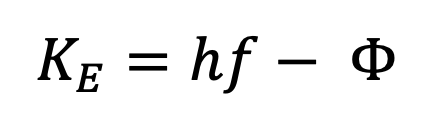The voltage (in eV) required to stop the electric current resulted from the photoelectric effect is given by: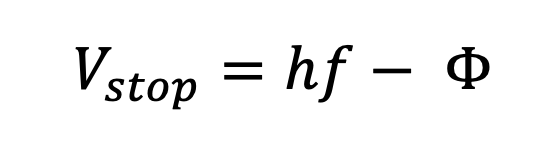• If the electron is some distance into the material of the cathode (away from the surface), some energy will be lost as it moves towards the surface.
• This means the final kinetic energy possess by electrons ejected from deep down in the cathode will be lower.
• The most energetic electrons (greatest kinetic energy) emitted will be those very close to the surface.

How does Einstein’s particle model of radiation explain the photoelectric effect?

• The main implication of Einstein’s theory is that he provided an explanation for the independence on intensity. In other words, no matter how intense or bright the incident radiation or light is, the energy of ejected electrons is not affected.
• More importantly, if the frequency of a quantum is lower than the work function of a material, photoelectric effect cannot occur regardless of radiation intensity.
• However, greater intensity means more quanta can transfer energy to more electrons. More electrons for a given voltage leads to greater number of electrons passing through the ammeter per unit time. So, current increases with intensity (if the work function is met).Graphing photoelectric effect results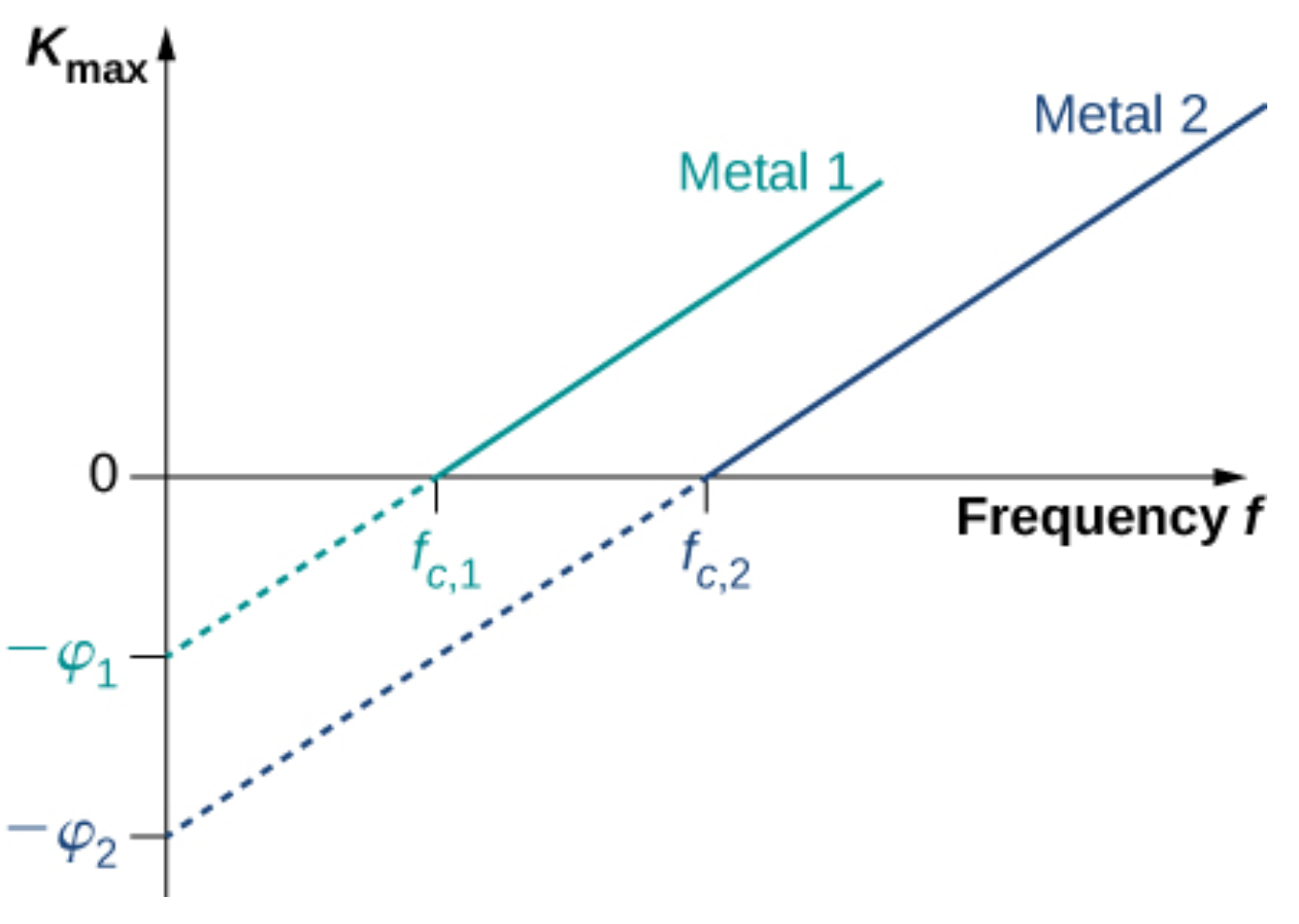• When electrons’ kinetic energy (y-axis) is plotted as a function of frequency (x-axis)
• the slope represents Planck’s constant h
• the y-intercept represents the negative value of work function.
• the x-intercept represents the threshold frequency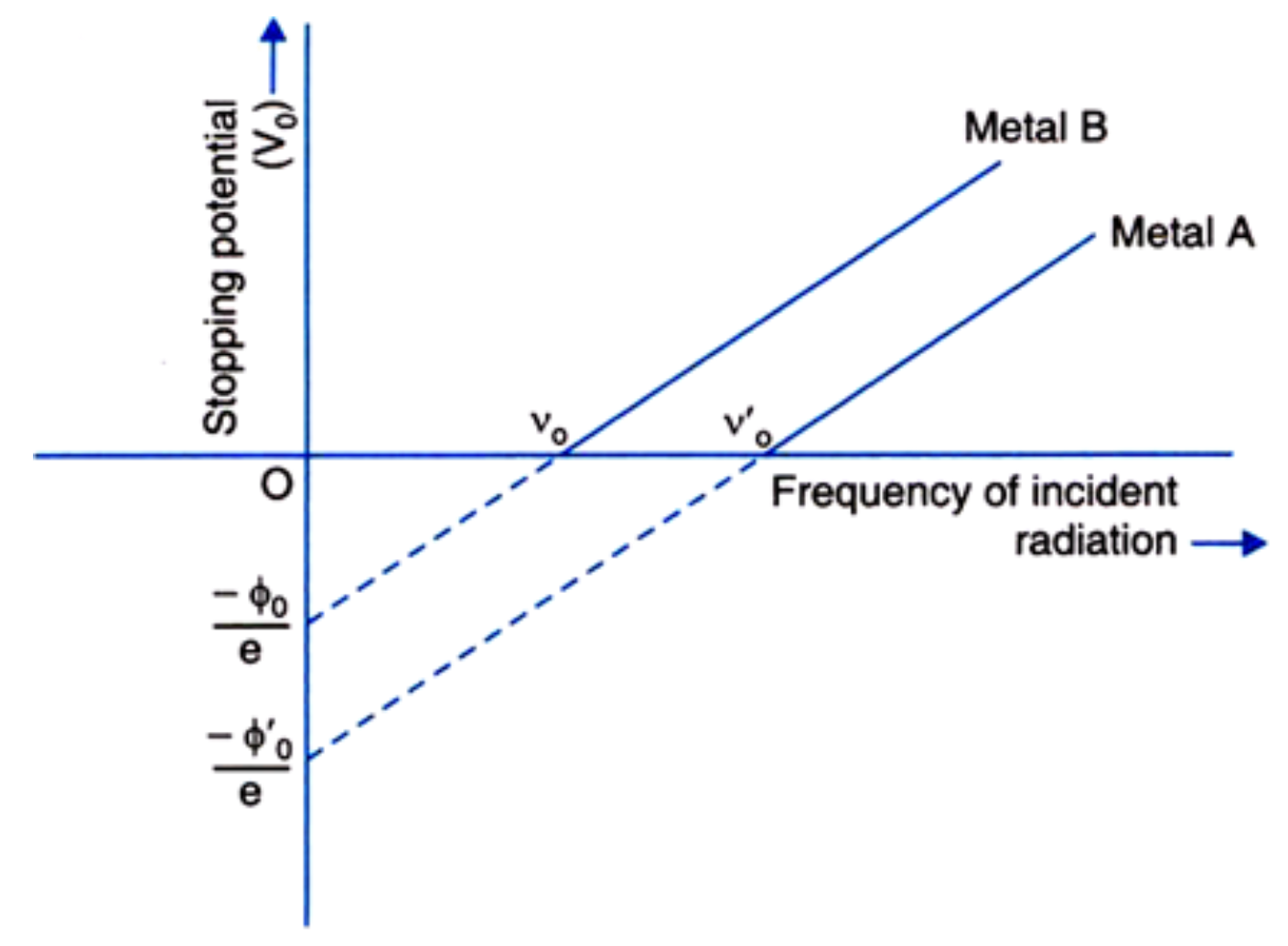• When stopping voltage (y-axis) is plotted as a function of frequency (x-axis)
• stopping voltage is usually measured in electron volts (eV), this needs to be converted into joules (SI unit for energy)

From the reference sheet:• the slope represents h/e (ratio between Planck’s constant and electron charge)
• the y-intercept represents -Φ/e (ratio between negative value of work function and electron charge)

Practice Question 1

What is the maximum kinetic energy of electrons ejected from calcium by 420 nm violet light, given that the work function for calcium metal is 2.71 eV? (2 marks)

Practice Question 2

The table shows work function of various metals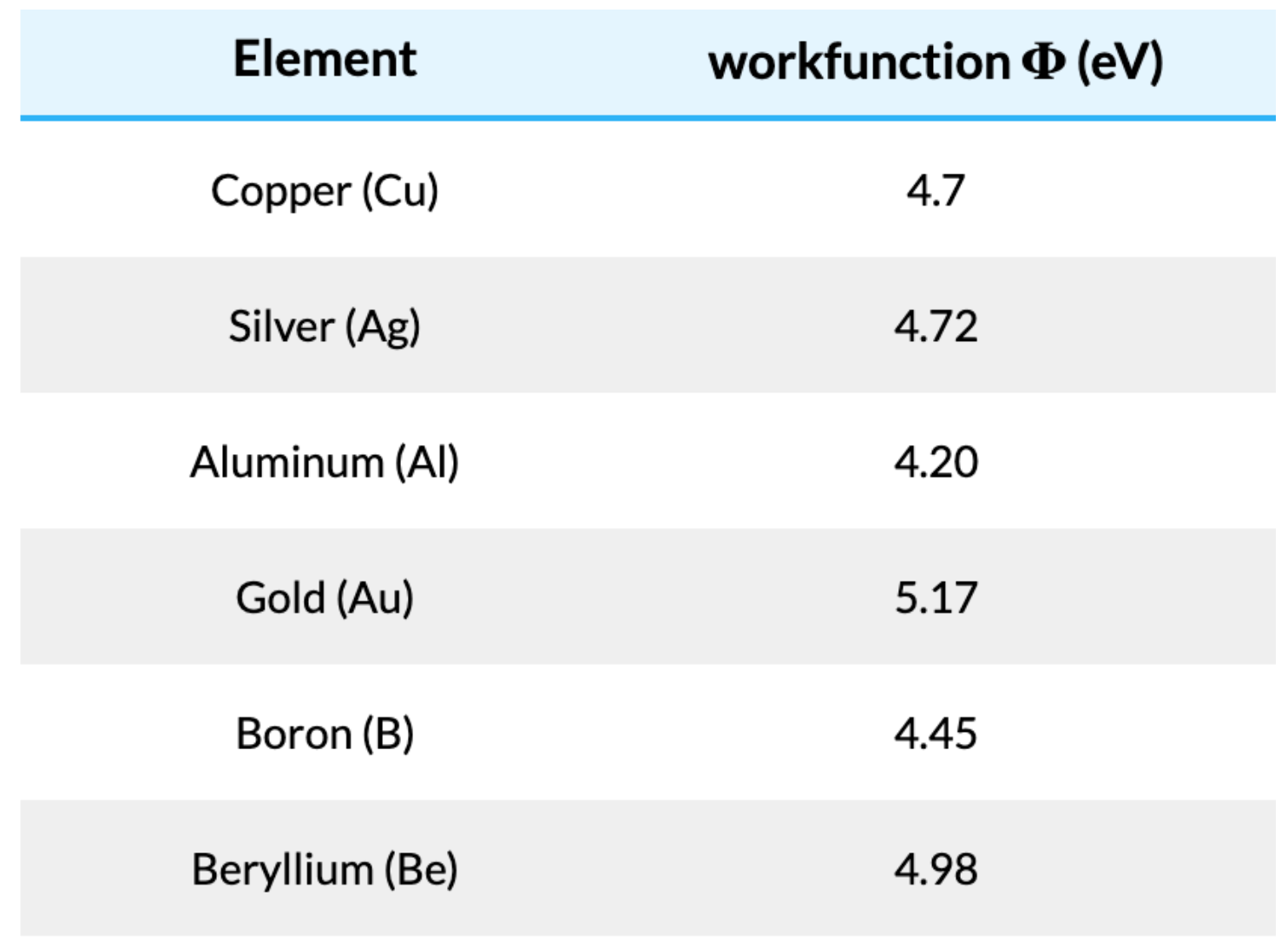What is the longest-wavelength electromagnetic radiation that can eject a photoelectron from silver? Is this in the visible range? (2 marks)

Previous section: The Quantum Theory and Wien's Displacement Law

Next section: Theory of Special Relativity: Einstein's Postulates